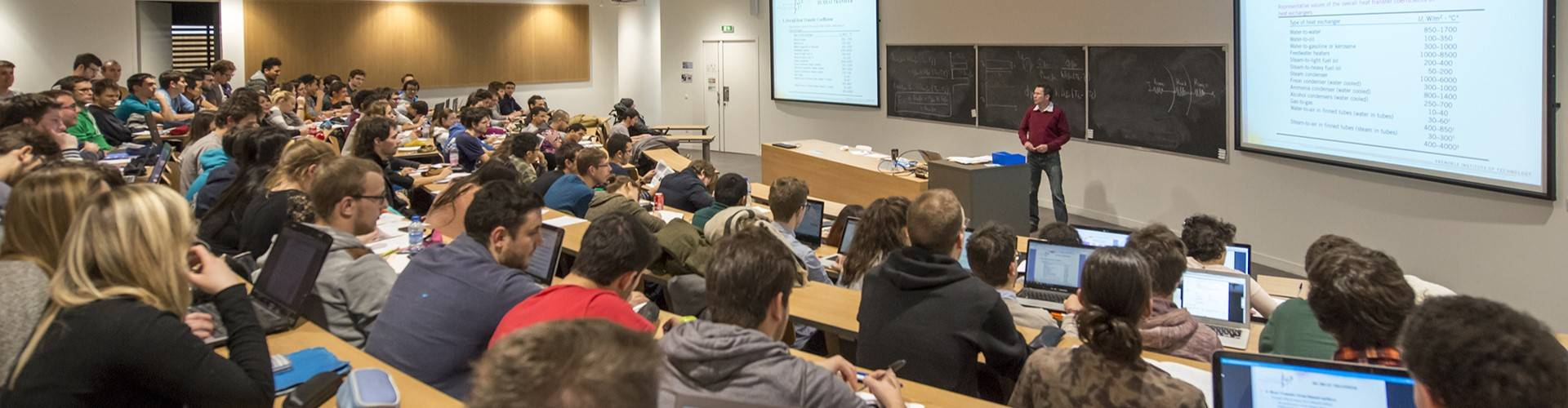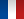# Signal and Image Processing - 4EUS4TSI

• #### Number of hours

• Lectures 30.0
• Projects -
• Tutorials -
• Internship -
• Laboratory works 30.0

ECTS 5.0

## Goal(s)

The purpose of this module is to go beyond the basic one-dimensional and deterministic approach of signals, providing theoretical and practical foundations for analysing and processing:

• random signals (spectral analysis and optimal filtering),
• images (foundations of image processing by covering both basic and more advanced topics illustrated on some real examples)

Responsible(s)

Florent CHATELAIN

## Content(s)

Image processing

• Definition of a digital image
• Color theory and color spaces
• Point-wise image transformations with applications in image enhancement
• Linear and non-linear image filtering
• Representation and processing of images in the Fourier domain
• Edge detection
• Mathematical morphology
• Image segmentation
• Image processing based on machine/deep learning

Spectral analysis

• Non parametric (or Fourier) spectral analysis
• Definition of the power spectral density
• Estimation of the auto-correlation function, bias and variance
• Correlogram (Balckman-Tuckey) : bias and variance trade-off, normalization, application
• Welch's periodogram, bias and variance trade-off, normalization
• Parametric spectral analysis
• Presentation of the approach, usefulness and interest
• Interpretation of the spectral estimator by ARMA(p,q) model, link with peaks and valleys of the PSD
• AR estimation
• ARMA estimation
• High resolution methods (optional)
• Prony
• CAPON
• MUSIC
• Application (lab): analysis of the vibration pollution of an industrial system

Optimal filtering

• Wiener filtering
• Principles, assumptions, Wiener-Hopf equation
• Non-causal optimal filtering
• Optimal causal filtering: Bode-Shannon decomposition
• Continuous and discrete time filters (IIR)
• Discrete Wiener filter
• Wiener-Hopf equation of the FIR filter
• Linear prediction, coding (analysis and synthesis) and AR parametric modeling
• Applications: periodic noise denoising (lab), LPC vocoder
• Assumptions, notion of recursion (prediction/correction structure) and adaptivity (example of the estimator of a mean)
• Applications: echo cancellation in audio systems, estimation of foetal ECG (lab: Widrow's experiment)

Prerequisites

• Mathematics for engineers
• complex variable functions,
• Fourier transform,
• Laplace transform,
• Z transform.
• Basics in continuous-time signal processing
• deterministic and random signals,
• time domain and frequency domain representations,
• linear and time-invariant filters, modulation,
• sampling.
• Basics in discrete-time signal processing
• discrete Fourier transform,
• analysis and design of digital filters.

Test

First session

• ER assessment : 2 hours supervised written + Image processing project
• EN assessment : work assignments + Lab reports
If distant learning mandatory:
• ER assessment : 2 hours homework + Image processing project and lab reports
• EN assessment : work assignments + other Lab reports

---------------

Second session

• EN assessment: retaking this assessment is not possible

ER 67% + EN 33%

Calendar

The course exists in the following branches:

see the course schedule for 2023-2024

Course language(s):